# Object Detection with Transformers

A complete guide to Facebook’s Detection Transformer (DETR) for Object Detection.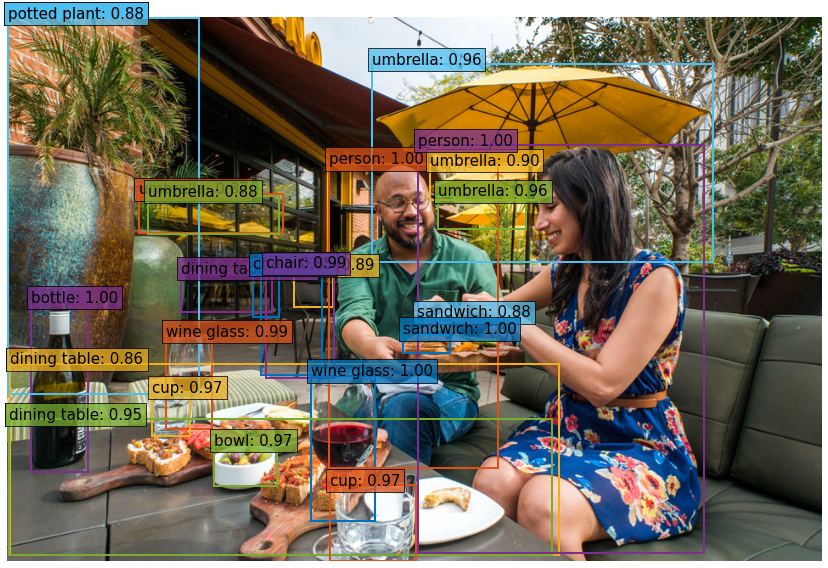Example output of DETR (source)

# Introduction

The DEtection TRansformer (DETR) is an object detection model developed by the Facebook Research team which cleverly utilizes the Transformer architecture. In this post, I’ll go through the inner-workings of DETR’s architecture to help provide some intuition on it’s moving parts. The colab notebook accompanying this tutorial can be found here:

Below, I’ll explain some of the architecture, but if you just want to see how to use the model, feel free to skip to the coding section.

# The Architecture

The DETR model consists of a pretrained CNN backbone (like ResNet), which produces a set of lower dimensional set of features. These features are then formatted into a single set of features of and added to a positional encoding, which is fed into a Transformer consisting of an Encoder and a Decoder in a manner quite similar to the Encoder-Decoder transformer described in the original Transformer paper (http://arxiv.org/abs/1706.03762). The output of the decoder is then fed into a fixed number of Prediction Heads which consist of a predefined number of feed forward networks. Each output of one of these prediction heads consists of a class prediction, as well as a predicted bounding box. The loss is calculated by computing the bipartite matching loss.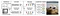DETR Architecture; from https://arxiv.org/pdf/2005.12872v3.pdf

The model makes a predefined number of predictions, and each of the predictions are computed in parallel.

## The CNN Backbone

Assume that our input image xᵢₘ of height H₀, width W₀, and three input channels. CNN backbone consists of a (pretrained) CNN (usually ResNet), which we use to generate C lower dimensional features having width W and height H (In practice, we set C=2048, W=W₀/32 and H=H₀/32).

This leaves us with C two-dimensional features, and since we will be passing these features into a transformer, each feature must be reformatted in a way that will allow the encoder to process each feature as a sequence. This is done by flattening the feature matrices into an H⋅W vector, and then concatenating each one.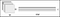Output of Convolutional Layer → Image Features

The flattened convolutional features are added to a spatial positional encoding which can either be learned, or pre-defined.

## The Transformer

The transformer is nearly identical to the original encoder-decoder architecture. The difference is that each decoder layers decodes each of the N (the predefined number of) objects in parallel. The model also learns a set of N object queries which are (similar to the encoder) learned positional encodings.

## Object Queries

The figure below depicts how N=20 learned object queries (referred to as prediction slots) focus on different areas of an image.

“We observe that each slot learns to specialize on certain areas and box sizes with several operating modes.” — The DETR Authors

An intuitive way of understanding the object queries is by imagining that each object query is a person. And each person can ask the, via attention, about a certain region of the image. So one object query will always ask about what is in the center of an image, and another will always ask about what is on the bottom left, and so on.

# Simple DETR Implementation with PyTorch

`import torchimport torch.nn as nnfrom torchvision.models import resnet50class SimpleDETR(nn.Module):"""Minimal Example of the Detection Transformer model with learned positional embedding""" def __init__(self, num_classes, hidden_dim, num_heads,             num_enc_layers, num_dec_layers):    super(SimpleDETR, self).__init__()    self.num_classes = num_classes    self.hidden_dim = hidden_dim    self.num_heads = num_heads    self.num_enc_layers = num_enc_layers    self.num_dec_layers = num_dec_layers    # CNN Backbone    self.backbone = nn.Sequential(         *list(resnet50(pretrained=True).children())[:-2])    self.conv = nn.Conv2d(2048, hidden_dim, 1)    # Transformer    self.transformer = nn.Transformer(hidden_dim, num_heads,         num_enc_layers, num_dec_layers)    # Prediction Heads    self.to_classes = nn.Linear(hidden_dim, num_classes+1)    self.to_bbox = nn.Linear(hidden_dim, 4)    # Positional Encodings    self.object_query = nn.Parameter(torch.rand(100, hidden_dim))    self.row_embed = nn.Parameter(torch.rand(50, hidden_dim // 2)    self.col_embed = nn.Parameter(torch.rand(50, hidden_dim // 2)) def forward(self, X):    X = self.backbone(X)    h = self.conv(X)    H, W = h.shape[-2:]    pos_enc = torch.cat([          self.col_embed[:W].unsqueeze(0).repeat(H,1,1),          self.row_embed[:H].unsqueeze(1).repeat(1,W,1)],       dim=-1).flatten(0,1).unsqueeze(1)    h = self.transformer(pos_enc + h.flatten(2).permute(2,0,1),    self.object_query.unsqueeze(1))    class_pred = self.to_classes(h)    bbox_pred = self.to_bbox(h).sigmoid()        return class_pred, bbox_pred`

## Bipartite Matching Loss (Optional)

Let ŷ={ŷ| i=1,…N} be the set of predictions where ŷ=(ĉᵢ, bᵢ) is the tuple consisting of the predicted class (which can be the empty class) and a bounding box bᵢ=(x̄, y̅, wᵢ, h) where the bar notation represents the midpoint between endpoints, and wᵢ and hᵢ are the width and height of the box, respectively.

Let y denote the ground truth set. Suppose that the loss between y and ŷ is L, and the loss between each yᵢ and ŷᵢ is L. Since we are working on the level of sets, the loss L must be permutation invariant, meaning that we will get the same loss regardless of how we order the predictions. Thus, we want to find a permutation σ∈ Sₙ which maps the indices of the predictions to the indices of the ground truth targets. Mathematically, we are solving for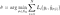Optimal Bipartite Matching

The process of computing σ_hat is called finding an optimal bipartite matching. This can be found using the Hungarian Algorithm. But in order to find the optimal matching, we need to actually define a loss function which computes the matching cost between yᵢ and ŷ_σ(i).

Recall that our predictions consist of both a bounding box and a class. Let’s now assume that the class prediction is actually a probability distribution over the set of classes. Then the total loss for the i-th prediction will be the loss that is generated from class prediction and the loss generated from the bounding box prediction. The authors http://arxiv.org/abs/1906.05909 define this loss as the difference in the bounding box loss and the class prediction probability:Matching Loss

where p-hatᵢ(cᵢ) is the argmax of the logits from cᵢ and Lbox is the loss resulting from the bounding box prediction. The above also states that the match loss is 0 if cᵢ=∅.

The box loss is computed as a linear combination of the L₁ loss (displacement) and the Generalized Intersection-Over-Union (GIOU) loss between the predicted and ground truth bounding box. Also, if you imagine two bounding boxes which don’t intersect, then the box error will not provide any meaningful context (as we can see from the definition of the box loss below).Box Loss

Where in the above equation the parameters λᵢₒᵤ and λ_L₁ are scalar hyperparameters. Notice that this sum is also a combination of errors generated from area and distance. Why does this make sense?

It makes sense to think of equation above as the total cost associated with the prediction b-hat_σ(i) where the price of area errors is λᵢₒᵤ and the price of distance errors is λ_L₁

Now let’s actually define the GIOU loss function. It is defined as follows:GIOU Loss

Since we are predicting classes from a given number of known classes, then class prediction is a classification problem, and thus we can use cross entropy loss for the class prediction error. We define the Hungarian loss function as the the sum of each N prediction losses:Hungarian Loss Function

# Using DETR for Object Detection

Here, you can learn how to load the pre-trained DETR model for object detection with PyTorch.

## Loading the Model

First import the required modules that will be used.

`# Import required modulesimport torchfrom torchvision import transforms as T import requests # for loading images from webfrom PIL import Image # for viewing imagesimport matplotlib.pyplot as plt`

The following code loads the pretrained model from torch hub with ResNet50 as a CNN backbone. For other backbones, see the DETR github

`detr = torch.hub.load('facebookresearch/detr',                      'detr_resnet50',                       pretrained=True)`

## Loading an Image

To load an image from the web, we use the requests library:

`url = 'https://www.tempetourism.com/wp-content/uploads/Postino-Downtown-Tempe-2.jpg' # Sample imageimage = Image.open(requests.get(url, stream=True).raw) plt.imshow(image)plt.show()`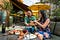Sample image. Source

# Setting up the Object Detection Pipeline

To input the image into the model, we need to convert the image from a PIL Image into a tensor, which is accomplished by using torchvision’s transforms library.

`transform = T.Compose([T.Resize(800),                       T.ToTensor(),                       T.Normalize([0.485, 0.456, 0.406],                                  [0.229, 0.224, 0.225])])`

The above transform resizes the image, converts the image from a PIL Image, and normalizes the image with mean-standard deviation. Where [0.485, 0.456, 0.406] are the means for each color channel, and [0.229, 0.224, 0.225] are the standard deviations for each color channel. To see more image transforms, see the torchvision documentation.

The model that we have loaded was pre-trained on the COCO Dataset with 91 classes along with an additional class representing the empty class (no object). We manually define each label with the following code:

`CLASSES = ['N/A', 'Person', 'Bicycle', 'Car', 'Motorcycle', 'Airplane', 'Bus', 'Train', 'Truck', 'Boat', 'Traffic-Light', 'Fire-Hydrant', 'N/A', 'Stop-Sign', 'Parking Meter', 'Bench', 'Bird', 'Cat', 'Dog', 'Horse', 'Sheep', 'Cow', 'Elephant', 'Bear', 'Zebra', 'Giraffe', 'N/A', 'Backpack', 'Umbrella', 'N/A', 'N/A', 'Handbag', 'Tie', 'Suitcase', 'Frisbee', 'Skis', 'Snowboard', 'Sports-Ball', 'Kite', 'Baseball Bat', 'Baseball Glove', 'Skateboard', 'Surfboard', 'Tennis Racket', 'Bottle', 'N/A', 'Wine Glass', 'Cup', 'Fork', 'Knife', 'Spoon', 'Bowl', 'Banana', 'Apple', 'Sandwich', 'Orange', 'Broccoli', 'Carrot', 'Hot-Dog', 'Pizza', 'Donut', 'Cake', 'Chair', 'Couch', 'Potted Plant', 'Bed', 'N/A', 'Dining Table', 'N/A','N/A', 'Toilet', 'N/A', 'TV', 'Laptop', 'Mouse', 'Remote', 'Keyboard', 'Cell-Phone', 'Microwave', 'Oven', 'Toaster', 'Sink', 'Refrigerator', 'N/A', 'Book', 'Clock', 'Vase', 'Scissors', 'Teddy-Bear', 'Hair-Dryer', 'Toothbrush']`

If we want to output different colored bounding boxes, we can manually define the colors we want in RGB format

`COLORS = [    [0.000, 0.447, 0.741],     [0.850, 0.325, 0.098],     [0.929, 0.694, 0.125],    [0.494, 0.184, 0.556],    [0.466, 0.674, 0.188],    [0.301, 0.745, 0.933]  ]`

## Formatting the Output

We also need to reformat the output of our model. Given a transformed image, the model will output a dictionary consisting of the probability of 100 predicted classes, and 100 predicted bounding boxes.

Each bounding box has the form `(x, y, w, h)` where (x,y) is the center of the bounding box (where the box is the unit square [0,1] ×[0,1]), and w, h are the widths and height of the bounding box. So we need to convert the bounding box output into the initial and final coordinates, and rescale the box to fit the actual size of our image.

The following function returns the bounding box endpoints:

`# Get coordinates (x0, y0, x1, y0) from model output (x, y, w, h)def get_box_coords(boxes):    x, y, w, h = boxes.unbind(1)    x0, y0 = (x - 0.5 * w), (y - 0.5 * h)    x1, y1 = (x + 0.5 * w), (y + 0.5 * h)    box = [x0, y0, x1, y1]    return torch.stack(box, dim=1)`

We also need to scale the box size. The following function does this for us:

`# Scale box from [0,1]x[0,1] to [0, width]x[0, height]def scale_boxes(output_box, width, height):    box_coords = get_box_coords(output_box)    scale_tensor = torch.Tensor(                 [width, height, width, height]).to(                 torch.cuda.current_device())    return box_coords * scale_tensor`

Now we need a function to encapsulate our object detection pipeline. The `detect`function below does this for us.

`# Object Detection Pipelinedef detect(im, model, transform):    device = torch.cuda.current_device()    width = im.size    height = im.size       # mean-std normalize the input image (batch-size: 1)    img = transform(im).unsqueeze(0)    img = img.to(device)        # demo model only support by default images with aspect ratio    between 0.5 and 2    assert img.shape[-2] <= 1600 and img.shape[-1] <= 1600,    # propagate through the model    outputs = model(img)    # keep only predictions with 0.7+ confidence    probas = outputs['pred_logits'].softmax(-1)[0, :, :-1]    keep = probas.max(-1).values > 0.85       # convert boxes from [0; 1] to image scales    bboxes_scaled = scale_boxes(outputs['pred_boxes'][0, keep], width, height)    return probas[keep], bboxes_scaled`

Now all we need to do to get our desired output is run the following:

`probs, bboxes = detect(image, detr, transform)`

# Plotting the Results

Now that we have our detected objects, we can use a simple function to visualize them

`# Plot Predicted Bounding Boxesdef plot_results(pil_img, prob, boxes,labels=True):    plt.figure(figsize=(16,10))    plt.imshow(pil_img)    ax = plt.gca()        for prob, (x0, y0, x1, y1), color in zip(prob, boxes.tolist(),   COLORS * 100):        ax.add_patch(plt.Rectangle((x0, y0), x1 - x0, y1 - y0,               fill=False, color=color, linewidth=2))        cl = prob.argmax()        text = f'{CLASSES[cl]}: {prob[cl]:0.2f}'        if labels:            ax.text(x0, y0, text, fontsize=15,                bbox=dict(facecolor=color, alpha=0.75))    plt.axis('off')    plt.show()`

Now we can visualize the results:

`plot_results(image, probs, bboxes, labels=True)`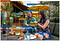Outputted results

# Final Thoughts

This post explained some of the details behind the Detection Transformer. Much of the code was inspired by the author’s tutorials which can be found here:

For more information on DETR, see the original author’s github page, and the article introducing the model:

## The Startup

Get smarter at building your thing. Join The Startup’s +725K followers.

Written by

## Jacob Briones

Specializing in explainable AI, mathematics, and physical sciences through the use of visualization, computer science, and creative writing.

## The Startup

Get smarter at building your thing. Follow to join The Startup’s +8 million monthly readers & +725K followers.

Written by

## Jacob Briones

Specializing in explainable AI, mathematics, and physical sciences through the use of visualization, computer science, and creative writing.## The Startup

Get smarter at building your thing. Follow to join The Startup’s +8 million monthly readers & +725K followers.

## More From Medium

Medium is an open platform where 170 million readers come to find insightful and dynamic thinking. Here, expert and undiscovered voices alike dive into the heart of any topic and bring new ideas to the surface. Learn more

Follow the writers, publications, and topics that matter to you, and you’ll see them on your homepage and in your inbox. Explore

If you have a story to tell, knowledge to share, or a perspective to offer — welcome home. It’s easy and free to post your thinking on any topic. Write on Medium